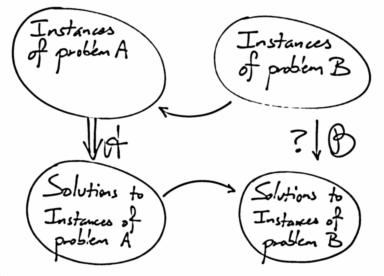# Problem A Is Harder Than Problem BAllPages RecentChanges Links to this page Edit this page Search Entry portal Advice For New Users

Prev: P Problems are in NP. / Next: Example NP Problems

# Comparing problem difficulty.

There are times when we don't really know how hard a problem is (whatever that might mean) but we do have a sense that one problem is harder than another. We might not know the absolute difficulty, but we might have a concept of the relative difficulty.

To help gain some intuition, here is a specific example ...

## Example: Factoring versus PrimalityThis diagram is left/right reversed from the other. Need to fix that.
As a specific example, suppose problem F is:

• Here's a number - give me its prime factorisation
• Given 3, return 
• Given 18, return [2, 3, 3]
• Given 70, return [2, 5, 7]
• Given 221, return [13, 17]
Suppose problem P is:
• Here's a number - is it prime?
• Given 3, return "Yes"
• Given 35, return "No"
• Given 11111111111, return "No"
• Given 11111111113, return "Yes"
 If you haven't already, it's worth reading the "What is a Problem?" page.
We can take a specific instance of problem P and convert it into an instance of problem F. Here's how:

• Instance of P:
• Here's a number N - is it prime?
becomes
• Instance of F:
• Here's a number N - give me the factorisation.
Assuming we can factorise, we can do that second step. Then we can convert the answer, the list of numbers, into an answer to the original question like this:

• Here's a list - is it of length 1?
So if you give me 1,111,111 and ask if it's prime, that's an instance of problem P. I then think of it as an instance of problem F. I can solve that by factorising it (giving the answer [239, 4649]) and then convert that into an answer to your original problem by saying: Is it of length 1?

 Unexpectedly, F seems to be strictly harder than P. We can test for primality with reasonable efficiency but we cannot (yet) factor effectively. See http://en.wikipedia.org/wiki/AKS_primality_test
In this case the answer is "No."

So 1111111 is not prime. Our ability to solve instances of problem F gives us the ability to solve instances of problem P. It's simple to see that this always works, and thus we have shown that factorising is at least as hard as determining whether a number is prime.

## Comparing problem difficulty generallySo now let's consider this more generally. What does it mean to say that Problem A "is harder than" Problem B?

Well, firstly, even though it's something of a mouthful, it's easiest to deal with the relationship "At least as hard as". This then allows that two problems are considered to be of equal difficulty. Even so, what does it mean to say that Problem A "is at least as hard as" Problem B?

We proceed on the idea that if problem A is harder (or at least as hard), then once we've solved problem A, solving problem B should be easier (or no harder).

So let's suppose we can solve problem A.

If we can now show that with a small amount of work we can convert an instance of problem B into an instance of problem A, and if we can also show that with another small amount of work that solution to that instance can then be converted back to a solution of the original instance, then that means that with a small amount of work we can solve B. We convert an instance of B to an instance of A, solve it, and then convert the solution back.

If we can do that, then assuming that we can solve problem A automatically implies that (with a "trivial" amount of work) we can solve B as well.

In that case we would have to consider problem B to be no harder than problem A.

## Observations

In the example we've been assuming that factoring is strictly harder than primality testing (unless P=NP). We have efficient ways to test if a number is prime, but we don't have efficient ways to factor. As a specific example of that, we know for sure that this number:

```7403756347956171282804679609742957314259
3188889231289084936232638972765034028266
2768919964196251178439958943305021275853
7011896809828673317327310893090055250511
6877063299072396380786710086096962537934
650563796359
```
is not prime, but no one (at the time of writing) knows its factors. You can find it and others here:
Prev: P Problems are in NP. / Next: Example NP Problems